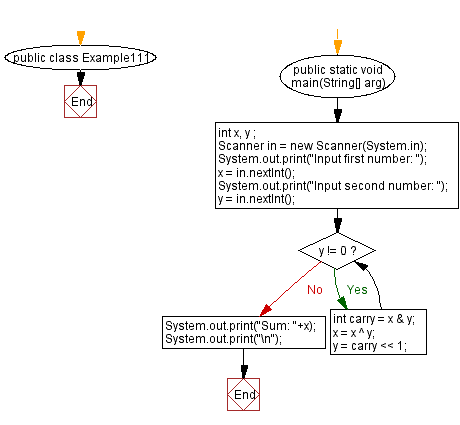﻿ Java: Add two numbers without using any arithmetic operators# Java Exercises: Add two numbers without using any arithmetic operators

## Java Basic: Exercise-111 with Solution

Write a Java program to add two numbers without using any arithmetic operators.

Given x = 10 and y = 12; result = 22

Sample Solution:

Java Code:

``````import java.util.Scanner;
public class Example111 {
public static void main(String[] arg)
{
int x, y ;
Scanner in = new Scanner(System.in);
System.out.print("Input first number: ");
x = in.nextInt();
System.out.print("Input second number: ");
y = in.nextInt();
while(y != 0){
int carry = x & y;
x = x ^ y;
y = carry << 1;
}
System.out.print("Sum: "+x);
System.out.print("\n");
}
}
```
```

Sample Output:

```Input first number: 10
Input second number: 12
Sum: 22
```

Pictorial Presentation:Flowchart:Java Code Editor:

What is the difficulty level of this exercise?

Test your Programming skills with w3resource's quiz.

﻿

## Java: Tips of the Day

getEnumMap

Converts to enum to Map where key is the name and value is Enum itself.

```public static <E extends Enum<E>> Map<String, E> getEnumMap(final Class<E> enumClass) {
return Arrays.stream(enumClass.getEnumConstants())
.collect(Collectors.toMap(Enum::name, Function.identity()));
}
```

Ref: https://bit.ly/3xXcFZt